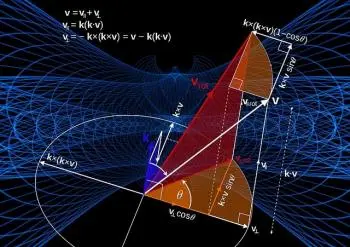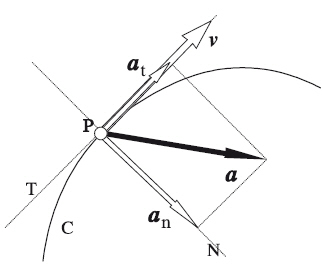# What Is Classical Physics? Concept and Branches of StudyClassical mechanics is a fundamental branch of physics that describes the motion of macroscopic objects in the world around us. It is a set of laws and principles that allow us to understand how objects at rest and in motion behave, from an apple falling from a tree to the complex motions of the planets in the solar system.

## What Are the Branches of Classical Physics?

The main branches of classical physics are:

1. Classical mechanics: It is the branch of physics that studies the movement of bodies and the forces that act on them. It includes concepts such as Newton's laws, kinematics and classical dynamics.

2. Classical fluid mechanics: It deals with the study of the behavior of fluids (liquids and gases) and the forces that act on them. Discuss topics such as fluid flow, pressure, viscosity, and aerodynamics.

3. Classical thermodynamics: Deals with the study of processes involving heat transfer and work. Classical thermodynamics focuses on macroscopic systems and the fundamental laws governing energy, temperature, and entropy.

4. Classical Electromagnetism: It is the study of electromagnetic interactions, including phenomena such as electricity, magnetism, electromagnetic waves, and classical optics. It is based on Maxwell's equations, which describe the relationship between electric and magnetic fields and their effects.

5. Classical optics: It deals with the study of light and its behavior in relation to objects and the media in which it propagates. Includes topics such as reflection, refraction, diffraction, and image formation.

6. Waves and acoustics: This branch focuses on the study of waves, including sound waves and their propagation in different media. It covers topics such as interference, diffraction, resonance, and superposition of waves.

## The Fundamentals of Classical Mechanics:

Classical mechanics is a fundamental branch of physics that focuses on the study of the motion of objects and the forces acting on them. It is a theory that is based on the principles developed by Sir Isaac Newton in the 17th century and has been widely applied and studied ever since.

Classical mechanics provides a conceptual and mathematical framework for describing and predicting the behavior of moving objects. Through concepts such as position, velocity, acceleration, and force, classical mechanics allows us to understand how objects move in space and how they respond to the forces that act on them.

The fundamental laws of classical mechanics are based on Newton's three laws.

### Newton's Laws

Newton's laws are the fundamental pillars of classical mechanics. These laws describe the behavior of moving objects and the relationship between the forces acting on them. Newton's three laws are:

• Newton's First Law or Law of Inertia: This law states that an object at rest will remain at rest and a moving object will continue to move at a constant speed in a straight line, unless an external force acts on it. In other words, objects tend to maintain their state of motion or rest unless a force is applied to them. This concept is known as the principle of inertia.

• Newton's second law or Law of force and acceleration: This law states that the acceleration of an object is directly proportional to the net force acting on it and inversely proportional to its mass. The equation that describes this law is F = ma, where F represents the net force, m is the mass of the object, and m is the acceleration it experiences.

• Newton's Third Law or Law of Action and Reaction: According to this law, for every action there is a reaction of equal magnitude but in the opposite direction. This means that when one object exerts a force on another, the second object also exerts a force of equal magnitude but in the opposite direction on the first. These forces are known as action-reaction pairs.

### Coordinate System and VectorsTo describe the motion of an object in classical mechanics, it is necessary to use a coordinate system and vector concepts. A coordinate system, such as the Cartesian system, is used to locate and describe the position of an object in three-dimensional space.

Vectors are mathematical tools that allow us to represent magnitudes that have both magnitude and direction in the three dimensions of space. In classical mechanics, vectors are used to describe the displacement, velocity, and acceleration of an object.

## Kinematics: the Movement of Objects

Kinematics deals with the study of the movement of objects without considering the causes that produce it. Kinematics allows us to understand and predict how objects move in space and how their position changes over time.

Through concepts such as trajectory, displacement, instantaneous velocity, and acceleration, we can accurately quantify and characterize the movement of objects.

### Displacement, Velocity, and Acceleration

Displacement refers to the change in position of an object relative to a reference point. It is represented by a vector that goes from the initial position to the final position. Displacement has both magnitude (distance) and direction.

Velocity is a measure of how quickly the position of an object changes in a given time interval. It is calculated by dividing the displacement by the elapsed time. Velocity is also a vector, which means it has magnitude (speed) and direction.

Acceleration represents the rate of change of an object's velocity as a function of time. Like displacement and velocity, acceleration is also a vector and can be positive (acceleration in the direction of movement) or negative (deceleration or acceleration in the opposite direction of movement).

### Uniform Rectilinear Motion (MRU)

In the context of classical kinematics, there are two main types of motion: uniform rectilinear motion (MRU) and uniformly accelerated rectilinear motion (MRUA).

The MRU refers to a movement in a straight line where the speed of the object is constant. In other words, there is no acceleration in the MRU. A common example of MRU is a car traveling at a constant speed on a straight, unobstructed road.

### Rectilinear Motion Uniformly Accelerated (MRUA)

On the other hand, Rectilinear Motion Uniformly Accelerated (MRUA) implies motion in a straight line where the velocity of the object changes constantly in time due to constant acceleration. An example of MRUA is a car accelerating from rest to a given speed in a specified time.

### Movement in Two and Three Dimensions:

In addition to motion in a straight line, classical mechanics also encompasses the study of motion in two and three dimensions. In this case, an object moves in a plane and can have motion components on both the horizontal (x) and vertical (y) axis. In the case of three-dimensional movements, a third axis (z) appears that indicates the depth.

The movement can be decomposed into different independent movements along each axis. This implies that the same kinematics equations can be applied to each motion component separately. Displacement, velocity, and acceleration in each direction are determined by vector calculations.

## Dynamics: Causes of Movement

Dynamics is the branch of classical mechanics that deals with the study of the causes of movement of objects. Unlike kinematics, which focuses on describing the properties of motion without considering forces, dynamics is concerned with understanding the interactions between objects and the forces acting on them.

In dynamics, the motion of objects is determined by Newton's laws mentioned above.

## Examples of Applications of Classical Mechanics

The theory of classical mechanics is crucial for the calculation of moving objects in fields such as industrial engineering, automotive or aeronautics.

Here are some typical application examples:

### Parabolic MovementClassical mechanics is widely applied in the study of projectile motion. The parabolic movement is the movement generated by a projectile launched with an inclination with respect to the horizontal that follows a parabolic trajectory due to the influence of gravity and air resistance.

By applying the concepts of classical kinematics and dynamics, it is possible to predict and analyze the range, maximum height, and velocity of a projectile based on its launch angle and initial velocity.

This type of analysis is fundamental in fields such as ballistics and sports (for example, in the parabolic shot in archery or the throwing of a ball in sports such as soccer or basketball).

### Circular Movement

An example of classical mechanics is the study of circular motion. In uniform circular motion (UCM), an object moves in a circular path with a constant velocity.

This type of movement is found in natural phenomena such as the orbit of the planets around the sun, the movement of the wheels of a vehicle, or even in amusement park attractions, such as Ferris wheels.

MCU analysis involves concepts such as angular velocity, period (the time required to complete one revolution), and centripetal acceleration, which is directed toward the center of the circle.

### Universal Law of Gravitation

The universal law of gravitation, formulated by Isaac Newton, is one of the most important contributions of classical mechanics. This law states that all objects in the universe are attracted to each other with a force that is directly proportional to the product of their masses and inversely proportional to the square of the distance that separates them.

The universal law of gravitation explains phenomena such as falling objects, the movement of the planets in their orbits around the sun, and the gravitational interaction between celestial bodies. It also allows you to calculate the gravitational force between two objects and predict how this force affects their movements.

The universal law of gravitation is essential in astronomy, astrophysics and space exploration, since it allows us to understand and predict the movements and interactions of celestial bodies in the universe.

Author:

Published: May 17, 2023
Last review: May 17, 2023